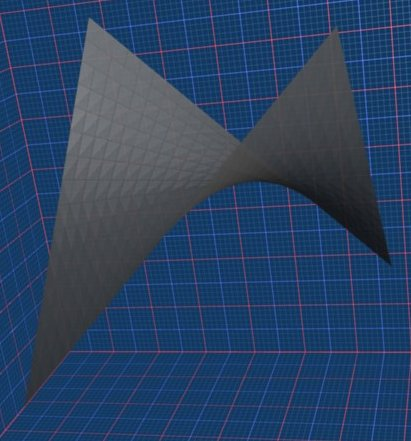# Maths - Elements of an equation

An equation is a mathematical expression with an equals sign in it.

Other possible elements of an equation are:

• numbers
• variables
• binary operators (+, -, * or ÷)
• unary operators (+ or -)
• brackets
• powers (xy)
• functions (sin(),log() , etc.)

These are explained below:

## Numbers

There are different types of numbers, for instance:

• Z- Integers - whole numbers: ... -3, -2, -1, 0, 1, 2, 3 ... both positive, zero and negative.
•- Real numbers - numbers which are continuous such as when we are representing points along a line - On this site I will sometimes use the term 'Scalar' to mean 'Real' numbers although strictly the term should be used when scaling a vector - In computer programs real numbers have a finite length and may have decimal point and/or exponent this allows us to approximate most real numbers but it is only an approximation.
• Q - Rational numbers - Integers and fractions where numerator and denominator are integers.
• Radical Integers - The integers plus any combination of addition, subtraction, multiplication, division and root extraction.
• Qalg - The root of a Z-polynomial - A complex number made up more than just radical integers although it is closed under sum, difference, product, quotient and nth root.
• Modulo 'n' numbers
• C - Complex Numbers - numbers with real and imaginary parts.
• H - Quaternion - Complex number whose elements are complex numbers.
• O - Octonion - Quaternion whose elements are complex numbers.
• Vectors - one dimensional arrays of numbers.
• Matrices - two dimensional arrays of numbers.

and these numbers may be coded in different ways:

• binary
• octal
• decimal

Unless otherwise specified we usually assume that numbers are decimal.

## Variables

Sometimes an equation contains a number but we don't yet know its value, or we may want to apply the equation to a range of values.

An example of the first is using x as the unknown, for example,

x + 1 = 3

An example of the second might be an equation of a line:

y = 2 * x + 3

In general we use:

x, y, z for unknowns.

a, b, c for values which are not yet specified.

## Binary Operators

The operators:

• - subtract
• * multiply (was x but for computers has to be changed to distinguish it from 24th letter of the alphabet)
• ÷ or / divide

take the two numbers on either side and replace it by a single number.

In the following plot the height is given by a+b, this gives a flat plane at 45° to both a and b:Of course the plane is infinite in all directions but here we have only shown a section of the plane.

### Multiplication

In the following plot the height is given by a*b, this gives a surface made up of straight lines along a or b directions (bi-linear). If we take a direction at 45° to both a and b then we get a parabola.how this graph was produced

## Unary operators

The operators:

• + plus
• - minus

apply to the number to the right. '-' inverts the number (subtracts from 0) '+' says the number to the right is positive (the default).

## Brackets

When we mix + and * then the answer we get depends on the order that we apply them.

For example

2 + 1 * 3

To clarify this we can put brackets around the operation to be applied first:

(2 + 1) * 3 = 9

2 + (1 * 3) = 5

If we don't specify which has precedence by using bracket then by default * and ÷ have precedence over + and -. So,

2 + 1 * 3 = 5

## Functions

A function takes one number and uses it to generate another number. For example the function sin() takes an angle as input and returns the ratio of opposite and hypotenuse in a right angled triangle.

 metadata block see also: Correspondence about this page Book Shop - Further reading. Where I can, I have put links to Amazon for books that are relevant to the subject, click on the appropriate country flag to get more details of the book or to buy it from them.The MathML Handbook - for people interested in working with mathematics on the web. Other Math Books Specific to this page here:

This site may have errors. Don't use for critical systems.